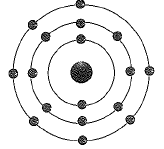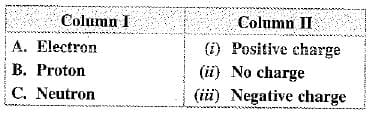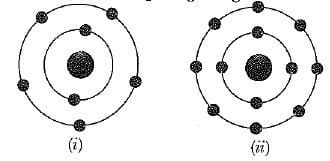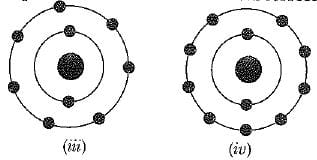# Test: Structure Of The Atom- 2

## 20 Questions MCQ Test Science & Technology for UPSC CSE | Test: Structure Of The Atom- 2

Description
Attempt Test: Structure Of The Atom- 2 | 20 questions in 20 minutes | Mock test for Class 9 preparation | Free important questions MCQ to study Science & Technology for UPSC CSE for Class 9 Exam | Download free PDF with solutions
QUESTION: 1

Solution:
QUESTION: 2

Solution:
QUESTION: 3

### In the Thomson’s model of atoms, which of the following statements are correct?  (i) The mass of the atoms is assumed to be uniformly distributed over the atom.  (ii) The positive charge is assumed to be uniformly distributed over the atoms.  (iii) The electrons are uniformly distributed in the positively charged sphere.  (iv) The electrons attract each other to stabilise the atom.

Solution:
QUESTION: 4

1 mole of diatomic element X2 contains 34 and 40 moles of electrons and neutrons respectively.
The isotopic formula of the element is

Solution:
QUESTION: 5

The diagram given below represent an atom ofSolution:
QUESTION: 6

The electronic configuration of Rb(37) will be

Solution:

Rubidium atoms have 37 electrons and the shell structure is 2,8,18,8,1.
The ground state electronic  configuration  of ground state gaseous neutral rubidium is
[ Kr].5 s1.

QUESTION: 7

Which of the following statements about Rutherford’s model of atoms are correct?
(i) Considered the nucleus as positively charged.
(ii) Established that the a-particles are four times as heavy as a hydrogen atom.
(iii) Can be compared to solar system.
(iv) Was in agreement with Thomson’s model.

Solution:
QUESTION: 8

Which of the following is correct?Solution:
QUESTION: 9

An atom with 3 protons and 4 neutrons will have a valency of

Solution:
QUESTION: 10

Which of the following are isobars?

Solution:
QUESTION: 11

The number of electrons in an element X is 15 and the number of neutrons is 16. Which of the following is the correct representation of the element?

Solution:
QUESTION: 12

Which of the following statement is always correct?

Solution:
QUESTION: 13

Rutherford’s a-scattering experiment led to the conclusion that

Solution:
QUESTION: 14

Which of the following pairs have identical values of charge/mass?

Solution:

Deuterium and an alpha particle have identical value of e/m.

QUESTION: 15

Which of the following in figures given below do not represent Bohr's model of an atom correctly ?Solution:

Figures (ii) and (iv) not correctly represent the Bohr's model of an atom.
It is because maximum number of electrons in K (I) shell is 2, not 4, so (ii) is wrong and maximum capacity of L (II) shell is 8 electrons, not 9. So, (iv) is also wrong.

QUESTION: 16

If K, L, M N, shells of an atom are full. The total number of electrons in that atom are:

Solution:

As we know, K shell contains 2 electrons  ; L she contains 8 electrons, M shell contains 18 electrons and N shell contains  32 electrons;  so total becomes
⇒ 60 electrons.

QUESTION: 17

Atomic models have been improved over the years. Arrange the following atomic models in the order of their chronological order.
(i) Rutherford’s atomic model
(ii) Thomson’s atomic model
(iii) Bohr’s atomic model

Solution:
QUESTION: 18

The electronic configuration of Ca(20) is

Solution:

The correct option is Option B.

Atomic number of calcium is 20. Number of protons = number of electrons = 20

First shell can accommodate 2 electrons so K=2.

Second shell can accommodate a maximum of 8 electrons so L=8.

Third shell can accommodate a maximum of 18 electrons but if we put the remaining 10 electrons it will violate the law that the outermost electron can accommodate 8 electrons so it will accommodate only 8 electrons.

So, the number of electrons in M is 8. Now the remaining two will be in N.

So the configuration is 2,8,8,2

QUESTION: 19

In Rutherford experiment, most of the alpha particles go straight through the foil because

Solution:

The particles of which most passed straight through the gold foil were alpha particles, which are positively charged. The few alpha particles that were deflected back or to one side were being repelled by nuclei, which are also positively charged. Those that passes straight through did so because they didn't encounter any nuclei. The observation that most alpha particles passed straight through the gold foil led Rutherford to conclude that the the positive charge in an atom in concentrated in a very small area, the nucleus
Atoms are mostly empty space. Electrons, which are negatively charged, are distributed throughout this space but take up a very small part of it

QUESTION: 20

Na+ has 12 neutrons and 10 electrons. Which of the following statement is correct?

Solution:

The atomic mass of an atom is the sum of the protons and neutrons in an atom.
Atomic mass = Protons + Neutrons ⇒ 11+12 ⇒ 23Use Code STAYHOME200 and get INR 200 additional OFF Use Coupon Code